Next: Kepler's Equation Up: Kepler's Equation Previous: Kepler's Equation

## The three anomalies

In the last section we showed that the orbits are ellipses following the equation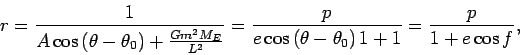(21)

In this equation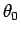is the angle of the semi-major axes with the ordinate,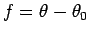is called the true anomaly. The angleis also commonly denoted by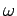. One focus sits at the origin of the coordinate system. The earth is presumed to be at this focus. The perigee is the point on the ellipse closest to this focus, the apogee, the point which is farthest. The true anomaly is the angle between a point on the ellipse and the perigee. Often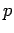is replaced by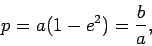where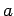is the semi-major and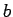the semi-minor axis.

It is often more useful to consider an ellipse in a coordinate system whose origin is at the center of the ellipse. The eccentric anomaly of a point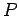on the ellipse is the angle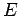shown in the picture below.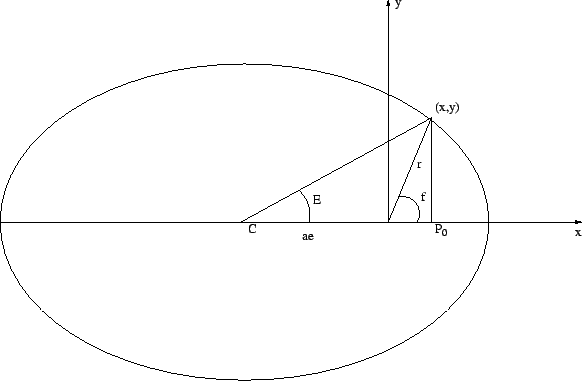We will next derive the connections between true anomaly and eccentric anomaly. Either angle can be used to describe the properties of an ellipse. In the above illustration, the pointhas coordinates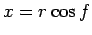and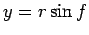, where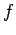is the true anomaly. The distance between the center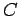and the focus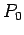is given by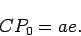Therefore, the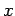-coordinate of the center is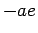. The-coordinate of the pointcan be computed usingorto get: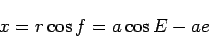For the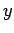-coordinate we observe: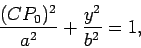i.e.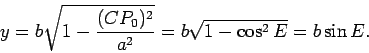To continue we see that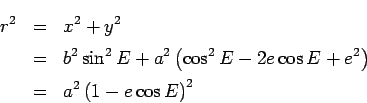Hence,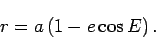To continue, we apply the double angle formula in the equation forto get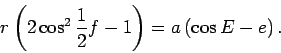Combining this with the equation for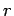in terms ofwe get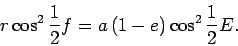In the same way we get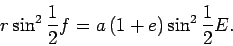Combining these we have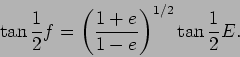(22)

A relation between the true and the eccentric anomaly.

The final angular measure to compute is the mean anomaly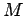. To do this we note that the satellite does not move with a constant angular velocity. If it were moving at a constant velocity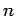then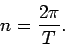Assuming that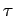is the time at which the satellite moves through the perigee, the mean anomaly at time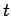is the angle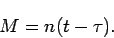The mean anomaly is specifically nice since it is a geometric quantity which is directly proportional to the time. To get the position of the satellite at a given time we need to compute either the eccentric or the true anomaly from the time. This relationship is called Kepler's equation.Next: Kepler's Equation Up: Kepler's Equation Previous: Kepler's Equation
Werner Horn 2006-06-06